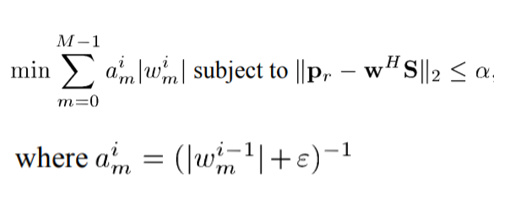# CVX error in using inv_pos

Hi,
I’m trying to run CVX to solve this problem:

cvx_begin
variable w1(n*L) complex
am=0;
w2=0;
for k=1:n
w_temp=w1((k-1)L+1:kL);
am=(norm(w_temp)+0.001);
w2=inv_pos(am)*norm(w_temp)+w2;
end
minimize(w2)
subject to
norm(pr-w1’*s)<=alpha
norm(L1’*w1)<=detal
cvx_end

And I have the following error:

Error using cvx/pow_cvx (line 144)
Disciplined convex programming error:
Illegal operation: pow_p( {convex}, {-1} )

Error in cvx/inv_pos (line 5)
y = pow_cvx( x, -1, ‘pow_p’ );


How can I construct this problem? Thank you for your reply.Your program violates CVX’s rules. As you can see in Why isn’t CVX accepting my model? READ THIS FIRST! your first responsibility is to show that your optimization problem is convex

Your problem Is not convex. Consider the special case in which w is a real scalar.variable, Then \|w\|/(\|w\|+\epsilon) is concave for any \epsilon > 0. Therefore, your function can not be convex.

1 Like

Did you perhaps find this in a paper which proposed a successive convex programming solution? For instance, start off with a starting value value (guess) for w. Then call CVX (convex solver) iteratively within a do while loop, each time replacing w in the denominator with its value form the previous iteration (starting value for first time though loop)? Each of these problems can easily be entered into CVX and solved, then w in the denominator is updated for the next iteration. I think there a problem along these lines posted to this forum some time ago.

There may not be a guarantee that this iterative scheme will converge to any answer, let alone a local minimum, let alone the global minimum. And its performance could heavily depend on the starting value chosen for w in the denominator…

1 Like

Thank you very much for you help, your advice is very relevant！I will try it.